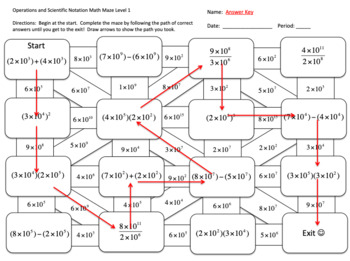# Operations and Scientific Notation Math MazeSubject
Resource Type
File Type

PDF

(1 MB|8 pages)
Product Rating
4.0
(2 Ratings)
Standards
• Product Description
• StandardsNEW

Students practice performing operations with scientific notation by completing a math maze! All answers in the maze are expressed in scientific notation.

They draw arrows to show the path that they took to get from the start of the maze to the exit.

This download contains three different mazes for teachers to use to differentiate their instruction.

Level 1 maze questions were designed for students who are struggling to pick up this concept. The questions used at this level contain smaller numbers. In addition, many numbers expressed in scientific notation are raised to the same power of ten.

Level 2 maze questions were designed for the average student. The questions used at this level have larger numbers. In addition, many numbers expressed in scientific notation are not raised to the same power of ten.

Level 3 maze questions were designed for stronger students as an enrichment activity. To correctly answer these problems, students must perform multiple operations.

If you like this math maze, you may also be interested in

Simplifying Algebraic Expressions Differentiated Math Maze

Solving Multi-Step Equations Differentiated Math Maze

Solving Multi-Step Inequalities Differentiated Math Maze

Solving Proportions Using Cross Products Differentiated Math Maze

Operations and Fractions Differentiated Math Maze

Calculating Slope Between Two Points Differentiated Math Maze

Exponential Properties Differentiated Math Maze

Solving Systems of Equations Differentiated Math Maze

Solving Absolute Value Equations Differentiated Math Maze

Solving Absolute Value Inequalities Differentiated Math Maze

Simplifying Radical Expressions Differentiated Math Maze

Multiplying Polynomials Differentiated Math Maze

Factoring Polynomials Differentiated Math Maze

Solving Polynomial Equations Differentiated Math Maze

Multiplying and Dividing Rational Expressions Differentiated Math Maze

Adding and Subtracting Rational Expression Differentiated Math Maze

For more work with operations and numbers in scientific notation, please consider purchasing the following products

Math Murder Mystery! Operations and Scientific Notation

Operations and Scientific Notation Jeopardy Game

Operations and Scientific Notation Scavenger Hunt

Operations and Scientific Notation Task Cards

**Finally, don't forget to rate and comment to earn TPT credits!**

Perform operations with numbers expressed in scientific notation, including problems where both decimal and scientific notation are used. Use scientific notation and choose units of appropriate size for measurements of very large or very small quantities (e.g., use millimeters per year for seafloor spreading). Interpret scientific notation that has been generated by technology.
Total Pages
8 pages
Included
Teaching Duration
30 minutes
Report this Resource to TpT
Reported resources will be reviewed by our team. Report this resource to let us know if this resource violates TpT’s content guidelines.